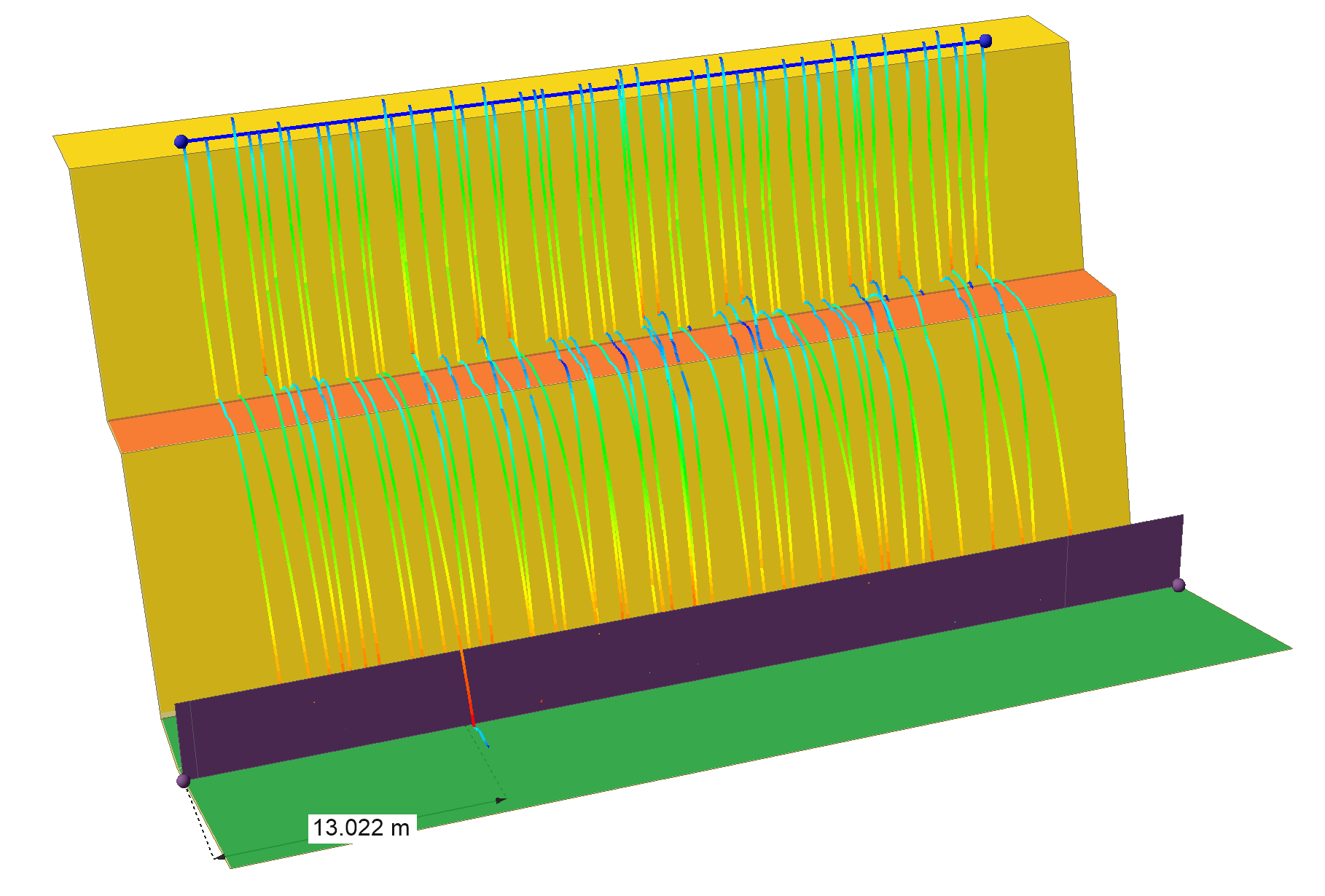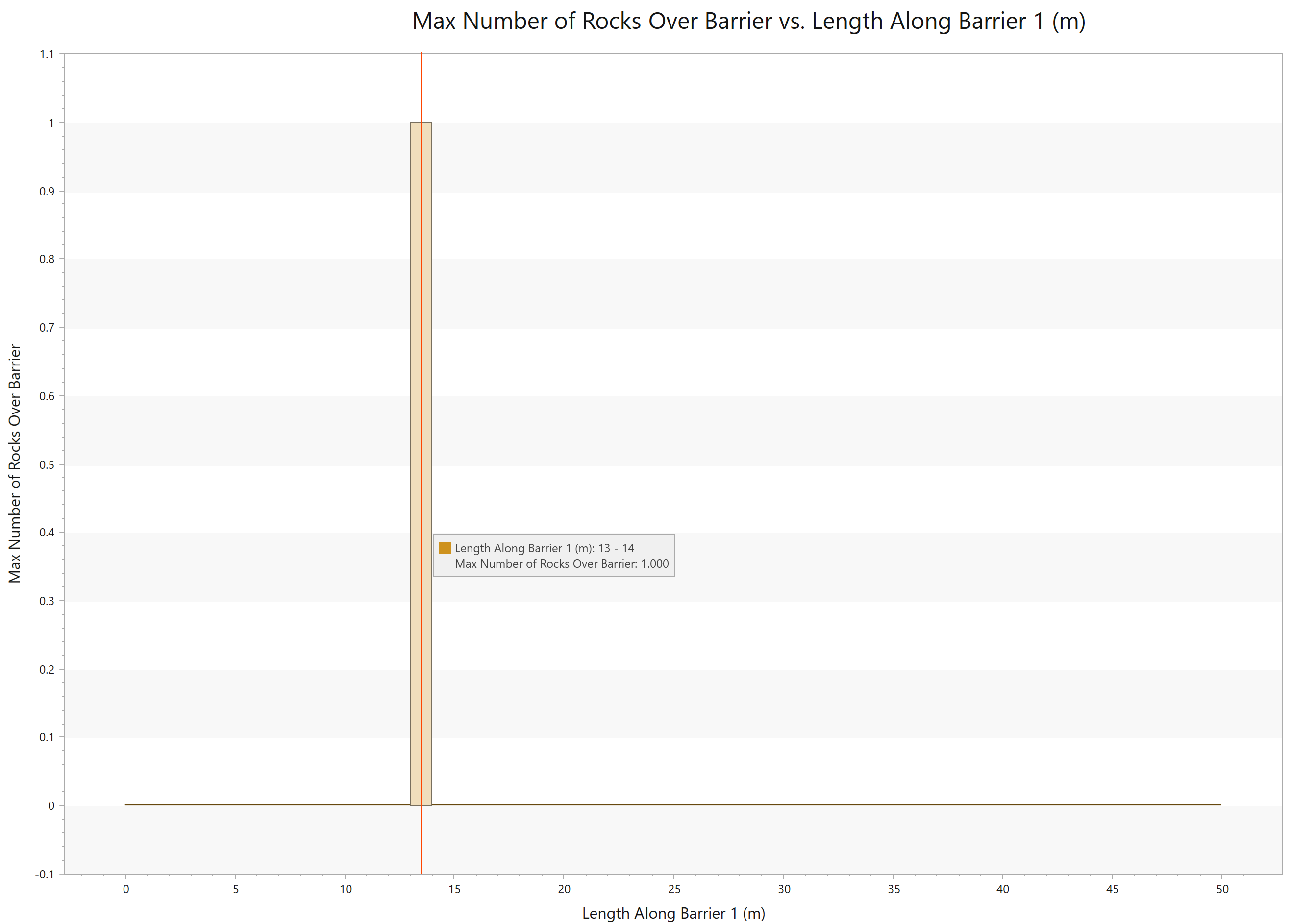Join one of our upcoming courses taking place around the world! Explore now

# Graph Along Barriers

RocFall3 allows users to graph rock impact results along a barrier's length by using the Graph Along Barriers tool. This tool is useful for understanding the most critical regions of a barrier.

Below is the list of result types that can be graphed along the length of each barrier:

• Total Kinetic Energy
• Translational Kinetic Energy
• Rotational Kinetic Energy
• Translational Velocity
• Rotational Velocity
• Impact Height
• Impact Angle
• Impact Angle (Absolute)
• Height Over Barrier
• Number of Hits
• Number of Rocks Over Barrier

To access the tool, the model must already have results computed and at least 1 barrier installed. Select Interpret > Graph Along Barriers to open the following dialog:

## Collector

In the Collector dropdown, the user selects the barrier of interest.

## Result Type

In the Results Type dropdown, the user may select the result type (e.g., height over barrier) to be graphed along the length of the selected barrier.

## Value to Plot

For result types of a continuous nature, the user may plot the Maximum value or a given percentile. In the Value to Plot dropdown, the user may specify this information by choosing Max or Percentile, respectively.

If the user elects to graph a percentile value, the specified value can be input in the percentage field to the right.

For result types of a discrete nature, the Value to Plot is always Frequency. An example of a result type of a discrete nature is the Number of Hits.

## Num Bins

The Num Bins field is used to specify the number of bins to be used for the graph. For example, if a barrier is 20 m in length, then a Num Bin value of 20 would segment the barrier into 1 m sections for graphing. A result is calculated for every bin based on the user selections for Result Type and Value to Plot.

As an example, in the following image, we see that one of the rock paths travelled over a barrier of 50 m length at approximately 13 m from one end of the barrier.A single rock path travelling over a barrier at approximately 13 m from one end of the barrier

If we graph the Number of Rocks Over Barrier with 50 bins, we see a frequency of 1, at bin 13 -14 m.Screenshot of the graph showing a frequency of 1 for 'Number of Rocks Over Barrier' at bin 13- 14 m.

Note that the reported frequencies, maximum values, and percentiles refer to calculations per bin. For example, if the result type is "total kinetic energy", and the value to plot is "max", then the graph shows the maximum total kinetic energy of rocks in each bin. Thus, it is important that the user establishes a meaningful bin size (e.g., a meaningful bin size could be the barrier width between posts).

The x-axis of this graph is the "length along barrier". As barriers are generated based on user drawing/input of vertices, the "start" of a barrier at 0 length along barrier is arbitrary. Therefore, to ensure the correct interpretation of where impacts are critical along a barrier, it is recommended to view this graph in conjunction with the barrier plot in 3D (Plot Barrier Results in 3D).